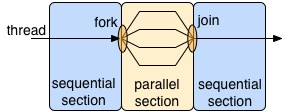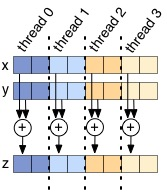## Lab 10

Computer Architecture I ShanghaiTech University
Lab 9 Lab 10 Lab 11

## Setup

Copy Makefile, hello.c, v_add.c and dotp.c to a suitable location in your home directory.

## Introduction to OpenMP

### Basics

OpenMP is a parallel programming framework for C/C++ and Fortran. It has gained quite a bit of traction in recent years, primarily due to its simplicity and good performance. In this lab we will be taking a quick peek at a small fraction of its features, but the links in the Additional Reference section can provide more information and tutorials for the interested.

There are many types of parallelism and patterns for exploiting it. OpenMP chooses to use a nested fork-join model. By default, an OpenMP program is a normal sequential program, except for regions that the programmer explicitly declares to be executed in parallel. In the parallel region, the framework creates (forks) a set number of threads. Typically these threads all execute the same instructions, just on different portions of the data. At the end of the parallel region, the framework waits for all threads to complete (join) before it leaves that region and continues sequentially.OpenMP uses shared memory, meaning all threads can access the same address space. The alternative to this is distributed memory, which is prevalent on clusters where data must be explicitly moved between address spaces. Many programmers find shared memory easier to program since they do not have to worry about moving their data, but it is usually harder to implement in hardware in a scalable way. Later in the lab we will declare some memory to be thread local (accessible only by the thread that created it) for performance reasons, but the programming framework provides the flexibility for threads to share memory without programmer effort.

### Hello World Example

For this lab, we will use C to leverage our prior programming experience with it. OpenMP is a framework with a C interface, and it is not a built-in part of the language. Most OpenMP features are actually directives to the compiler. Consider the following implementation of Hello World (hello.c):

```int main() {
#pragma omp parallel
{
}
}```

This program will fork off the default number of threads and each thread will print out "hello world" in addition to which thread number it is. The `#pragma` tells the compiler that the rest of the line is a directive, and in this case it is `omp parallel`. `omp` declares that it is for OpenMP and `parallel` says the following code block (what is contained in { }) can be executed in parallel. Give it a try:

```\$ make hello
\$ ./hello```

Notice how the numbers are not necessarily in numerical order and not in the same order if you run `hello` multiple times. This is because within a `omp parallel` region, the programmer guarantees that the operations can be done in parallel, and there is no ordering between the threads. It is also worth noting that the variable `thread_ID` is local to each thread. In general with OpenMP, variables declared outside an `omp parallel` block have only one copy and are shared amongst all threads, while variables declared within a `omp parallel` block have a private copy for each thread.

## Exercises

Vector addition is a naturally parallel computation, so it makes for a good first exercise. The `v_add` function inside v_add.c will return the array that is the cell-by-cell addition of its inputs `x` and `y`. A first attempt at this might look like:

```void v_add(double* x, double* y, double* z) {
#pragma omp parallel
{
for(int i=0; i<ARRAY_SIZE; i++)
z[i] = x[i] + y[i];
}
}```

You can run this (`make v_add` followed by `./v_add`) and the testing framework will automatically time it and vary the number of threads. You will see that this actually seems to do worse as we increase the number of threads. The issue is that each thread is executing all of the code within the `omp parallel` block, meaning if we have 8 threads, we will actually be adding the vectors 8 times. To get speedup when increasing the number of threads, we need each thread to do less work, not the same amount as before.Your task is to modify `v_add` so there is some speedup (speedup may plateau as the number of threads continues to increase). The best way to do this is to decrease the amount of work each thread does. To aid you in this process, two useful OpenMP functions are:

```int omp_get_num_threads();

The function `omp_get_num_threads()` will return how many threads there are in a `omp parallel` block, and `omp_get_thread_num()` will return the thread ID.

Divide up the work for each thread through two different methods (write different code for each of these methods):

• First, have each thread handle adjacent sums: i.e. Thread 0 will add the elements at index 0, Thread 1 will add the elements at index 1, etc. This method will not be very efficient. It will encounter the problem known as false sharing.
• Second, if there are N threads, break the vectors into N contiguous chunks, and have each thread only add that chunk (like the figure above).

For this exercise, we are asking you to manually split the work amongst threads. Since this is such a common pattern for software, the designers of OpenMP made the `for` directive to automatically split up independent work. Here is the function rewritten using it. You may NOT use this directive in your solution to this exercise (Exercise 1).

```void v_add(double* x, double* y, double* z) {
#pragma omp parallel
{
#pragma omp for
for(int i=0; i<ARRAY_SIZE; i++)
z[i] = x[i] + y[i];
}
}```

#### Checkoff

• Show the TA your code for `v_add` that manually splits up the work (both methods)
• Run your code to show that it gets parallel speedup (second method)

### Exercise 2: Dot Product

The next interesting computation we want to compute is the dot product of two vectors. At first glance, implementing this might seem not too dissimilar from `v_add`, but the challenge is how to sum up all of the products into the same variable (reduction). A sloppy handling of reduction may lead to data races: all the threads are trying to read and write to the same address simultaneously. One solution is to use a critical section. The code in a critical section can only be executed by a single thread at any given time. Thus, having a critical section naturally prevents multiple threads from reading and writing to the same data, a problem that would otherwise lead to data races. A naive implementation would protect the sum with a critical section, like (dotp.c):

```double dotp(double* x, double* y) {
double global_sum = 0.0;
#pragma omp parallel
{
#pragma omp for
for(int i=0; i<ARRAY_SIZE; i++)
#pragma omp critical
global_sum += x[i] * y[i];
}
return global_sum;
}```

Try out the code (`make dotp` and `./dotp`). Notice how the performance gets much worse as the number of threads goes up? By putting all of the work of reduction in a critical section, we have flattened the parallelism and made it so only one thread can do useful work at a time (not exactly the idea behind thread-level parallelism). This contention is problematic; each thread is constantly fighting for the critical section and only one is making any progress at any given time. As the number of threads goes up, so does the contention, and the performance pays the price. Can you fix this performance bottleneck?

First, try fixing this code without using the OpenMP Reduction keyword. Hint: Try reducing the number of times a thread must add to the shared `global_sum` variable.

Now, fix the problem using OpenMP's built-in Reduction keyword (Google for more information on it). Is your performance any better than in the case of the manual fix? Why?

#### Checkoff

• Show the TA your manual fix to `dotp.c` that gets speedup over the single threaded case
• Show the TA your Reduction keyword fix for `dotp.c`, and explain the difference in performance.